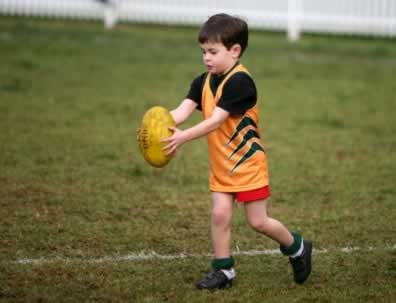﻿ ELLLO Views #278
Views # | Intermediate 4

# --------

-----------------------This is where the text goes. This is where the text goes. This is where the text goes. This is where the text goes. This is where the text goes. This is where the text goes.

This is where the text goes. This is where the text goes. This is where the text goes. This is where the text goes. This is where the text goes. This is where the text goes. This is where the text goes. This is where the text goes. This is where the text goes. This is where the text goes.

Learn vocabulary from the lesson!

word 1quote

Notice the following:

1. sample 1
2. sample 2

word 2quote

Notice the following:

1. sample 1
2. sample 2

word 3quote

Notice the following:

1. sample 1
2. sample 2

word 4quote

Notice the following:

1. sample 1
2. sample 2

word 5quote

Notice the following:

1. sample 1
2. sample 2

word 6quote

Notice the following:

1. sample 1
2. sample 2

1 • 2 • 3
4 • 5
1. ---- .
2. ---- .
3. ---- .
4. ---- .
5. ---- .